[BACK]
 Intelligent Automation & Soft ComputingDOI:10.32604/iasc.2021.016464Article

Slime Mold Optimizer for Transformer Parameters Identification with Experimental Validation

1Department of Electrical Engineering, College of Engineering, Taif University, P.O. Box 11099, Taif, 21944, Saudi Arabia
2Electrical Engineering Department, Faculty of Engineering, Al-Azhar University, Cairo, 11651, Egypt
3Electrical Engineering Department, Faculty of Engineering, Northern Border University, Arar, 1321, Saudi Arabia
*Corresponding Author: Salah K. Elsayed. Email: sabdelhamid@tu.edu.sa
Received: 03 January 2021; Accepted: 23 February 2021

Abstract: The problem of parameters identification for transformer equivalent circuit can be solved by optimizing a nonlinear formula. The objective function attempts to minimize the sum of squared relative errors amongst the accompanying calculated and actual points of currents, powers, and secondary voltage during the load test of the transformer subject to a set of parameters constraints. The authors of this paper propose applying a new and efficient stochastic optimizer called the slime mold optimization algorithm (SMOA) to identify parameters of the transformer equivalent circuit. The experimental measurements of load test of single- and three-phase transformers are entered to MATLAB code for extracting the transformer parameters through minimizing the objective function. Experimental verification of SMOA for parameter estimation of single- and three-phase transformers shows the capability and accuracy of SMOA in estimating these parameters. SMOA offers high performance and stability in determining optimal parameters to yield precise transformer performance. The results of parameters identification of transformer using SMOA are compared with the results using three optimization algorithms namely atom search optimizer, interior search algorithm, and sunflower optimizer. The comparisons are fairly performed in terms of the smallness of objective function. Comparisons shows that SMOA outperforms other contemporary algorithms at this task.

Keywords: Parameter extraction; transformer; equivalent circuit; slime mold algorithm

Nomenclature

 SMOA: slime mould optimization algorithm R1 : the resistance of the primary winding (Ω) X1 : the leakage reactance of primary winding (Ω) R2′ : the refereed resistance of the secondary winding (Ω) X2′ : the refereed leakage reactance of secondary winding (Ω) Rc : the core loss resistance (Ω) Xm : the magnetizing reactance (Ω) V1 : the primary voltage (V) V2′ : the refereed secondary voltage (V) Z1 : the impedance of the primary winding (Ω) Z2′ : the refereed impedance of the secondary winding (Ω) Zm : the magnetizing impedance (Ω) Z : the total transformer impedance (Ω) I1 : the input current (A) I2′ : the refereed secondary current (A) Io : the no-load current (A) P1 : the input power (W) P2 : the output power (W) η: the efficiency (%) SSRE: the sum of squared relative errors N: the number of measurements m: the measured values e : the estimated values Fobje : the objective function X : the location of the slime mould Xb→(k) : the candidate with the highest order concentration in the iteration k k: iteration number kmax : the maximum number of iterations XA and XB : two randomly selected candidates from the slime mould swarm υb : variable lies in the range [-a, a] υc : variable that linearly decreased from one to zero W : the slime mould weight bF : the best fitness in the current iteration wF : the worst fitness in the current iteration S(i) : the fitness of candidate X Bs : the best-obtained fitness throughout all iterations rand and r: and r random vector in the range of [0,1] Max and Min: the border limits of search space popu: population ASO: atomic search optimizer ISA: interior search algorithm SFO: sunflower optimizer

1  Introduction

Transformers are major components in power systems that are important in both transmission and distribution networks. They are also used in industrial and household devices. Transformer maloperation significantly affects system performance, reliability, and stability [1,2].

A transformer’s equivalent circuit has parameters for resistance and reactance that should be identified for accurate analysis of electric power grids. Transformer parameters also have a major effect on the transformer’s performance in different operating conditions. Accurately estimating transformer parameters arises from the need to improve transformer performance in both steady-state and transient operating conditions. Realistic studies in system behavior require a reasonable transformer model, which plays an important role in the integration between the other components .

A transformer is modeled by considering its nonlinearities [2,4]. Additionally, the presence of saturation, harmonics, and transient situations affects the estimation parameters of the transformer. Actual real-time measurements are needed to perform the time-domain [5,6] and frequency response [7,8] analyses when estimating accurate transformer parameters.

Generally, transformer parameters can be computed in various ways: standard test executions (no-load and short circuit tests) [9,10], geometrical dimensions of a transformer’s construction , data from product nameplates [12,13], and various load details [10,14]. Techniques using the geometrical dimensions of the transformer require data obtained from terminal measurements, external tank dimensions, and nameplates. Determining the cross-sectional area of both the yokes and the limb as well as the core dimensions requires solving system equations that are limited by the dimensions of the transformer tank . The standard no-load and short-circuit tests cannot be utilized when the transformers are in operation in the circuit. In addition to the defects of methods other than the load data method, estimating parameters using the measured load data values minimizes the difference between estimated and measured values .

Recent optimization techniques have made major strides in solving power problems such as optimal power flow , load frequency control [16,17], energy management , and parameter estimation for electrical instruments such as photovoltaic modules [19,20], and fuel cells . However, all these estimation methods use optimizers that compare estimated values with measured values to minimize deviations.

Other researchers have implemented numerous optimization techniques for identifying transformer parameters such as a chaotic optimization algorithm , imperialist competitive and gravitational search algorithms , an evolutionary programming algorithm , a bacterial foraging algorithm , particle swarm optimization , a genetic algorithm , an artificial bee colony algorithm , a coyote optimization algorithm , and a manta ray foraging optimization (MRFO) method and a chaotic variant . These algorithms can be implemented using load data or nameplate data for the transformer, while the transformer is in service (i.e., without disconnecting it). Finally, these algorithms can estimate transformer parameters in both single- and three-phase systems.

Despite this brief survey, the no-free-lunch theorem demonstrates that the possibility of further improvement in estimating transformer parameters remains. To this end, the authors of this paper consider using slime mold optimization algorithm (SMOA), which was created in 2020 to estimate unknown transformer parameters. SMOA was inspired as a novel meta-heuristic algorithm by slime mold oscillation modes in nature and applied effectively to the designs of pressure containers and the welded beams . In this paper, single- and three-phase transformers are investigated using load tests to demonstrate the effectiveness of SMOA and make essential comparisons. Our performance evaluations show that our proposed method outperforms existing approaches.

The main contribution of this paper can be summarized as follows:

• Application of SMOA to estimate transformer parameters.

• Experimentation of single- and three-phase transformers for validation of the proposed method.

• Comparison of SMOA with other optimization techniques based on their results.

The paper is organized as follows. In Section 2, introduce the equivalent circuit of a transformer. Section 3 gives a short overview of the SMOA method. Section 4 presents our experiment, the numerical results of the application of SMOA, and discussion. Finally, Section 5 introduces our conclusions.

2  Problem Formulation

The per-phase equivalent circuit of a three-phase power transformer with respect to its primary side is shown in Fig. 1. There are six parameters: R1,X1,R2,X2,Rc, and Xm .Figure 1: Per phase equivalent circuit of electric power transformer with respect to its primary side

Using the fundamental laws of electric circuits, the following equations can be formulated:

V1=E1+Z1I1 (1)

E1=ZmIo (3)

Z1=R1+jX1 (4)

Z2=R2+jX2 (5)

Zm=jXm.RcRc+jXm (6)

I1=V1Z1=Io+I2 (8)

Io=E1Zm=E1Rc+E1jXm=IcjIm (9)

P1=(V1I1) (11)

P2=(V2I2) (12)

η=P2P1 (13)

Minimizing the sum of squared relative errors (SSRE) amongst the estimated and measured points is the objective function (Fobje) for the transformer parameters. It is extracted as,

Fobje =min(SSRE)=min{ i=1N[ (I1e(i)I1m(i)1)2+( I2e (i)I 2m(i)1)2+(P 1e(i)P1m (i)1)2+(P2e(i)P2m(i)1)2+ (V2e(i)V2m(i)1) 2]} (14)

where Fobje is subject to constraints, which are defined by the lower and upper limits of the transformer parameters.

3  SMOA

SMOA is inspired by slime mold oscillation modes in nature. The slime mold that inspired this algorithm is physarum polycephalum, which is classified as a fungus and termed a slime mold by Howard. It is a eukaryote that lives in humid and cold places. The plasmodium is the main nutritional stage of slime mold where its organic matter pursues food, borders it, and excretes enzymes to predigest it. Throughout the migration stage, the front end of the slime mold spreads out like a fan-shaped tailed from a structured intravenous tie that lets cytoplasm stream inside. A distinctive feature of slime mold, it can exploit numerous sources of food simultaneously forming a venous network connecting them. They can even propagate to large areas, if the environment is suitable and food is sufficient .

The SMOA method has several features. It is characterized by a unique mathematical model. The adaptive weights permit the SMOA to maintain a specific perturbation rate and guarantee fast convergence that prevents the falling into local optima. It also displays exceptional exploratory and exploitative abilities. To establish the optimal route for obtaining food, SMOA uses adaptive weights to simulate a slime mold’s generation of positive and negative feedback as it spreads, a bio-oscillator created by its search for food. SMAO can also make correct decisions based on historical data due to its excellent utilization of individual fitness values. The description of SMOA model in mathematical terms is in the following paragraphs.

3.1 Food Approaching

The slime mold uses odors in the air to find food. Eq. (15) emulates this behavior in contraction mode.

X(k+1)={Xb(k)+νb(WXA(k)XB(k)),r<pνcX(k),rp (15)

and

p=tanh|S(i)BS|, (16)

where υb[a,a] ,

a=tanh1((kkmax)+1) (17)

W(SmellIndex(i))={1+rlog(bFS(i)bFwF+1),condition1rlog(bFS(i)bFwF+1),others. (18)

In these equations, the condition referring to S(i) is arranged in the first half of the population, and SmellIndex is the individual fitness ranked in descending order as stated in Eq. (19).

SmellIndex=sort(S) (19)

3.2 Food Wrapping

The location of the slime mold (X) is updated according to Eq. (20).

X={rand(MaxMin)+Min,rand<zXb(k)+νb(W.XA(k)XB(k)),r<pνcX(k),rp (20)

3.3 Oscillation

The value of vb changes randomly between [−a, a], and it steadily approaches zero as the iterations increase, while vc oscillates between [1,0] and approaches zero. Tab. 1 presents the SMOA.

4  Results with Discussion

The experiments were conducted with two test cases: a single-phase transformer rated at 300 VA, 230/2·115 V, and a three-phase transformer rated at 300 VA, 400/2·200 V. One primary winding and two secondary windings were in the single-phase transformer and in each phase of the three-phase transformer. The load test was carried out on the two transformers and used the measurements to calculate SSRE as Fobje to be minimized by SMOA for estimating transformers parameters. SMOA’s results are compared to those from ASO , ISA , and SFO . Our results were obtained using MATLAB-R2016b under Windows 10 running on a laptop with an Intel Core i7−4702MQ CPU at 2.2 GHz with 8 GB of RAM.

Table 1: SMOA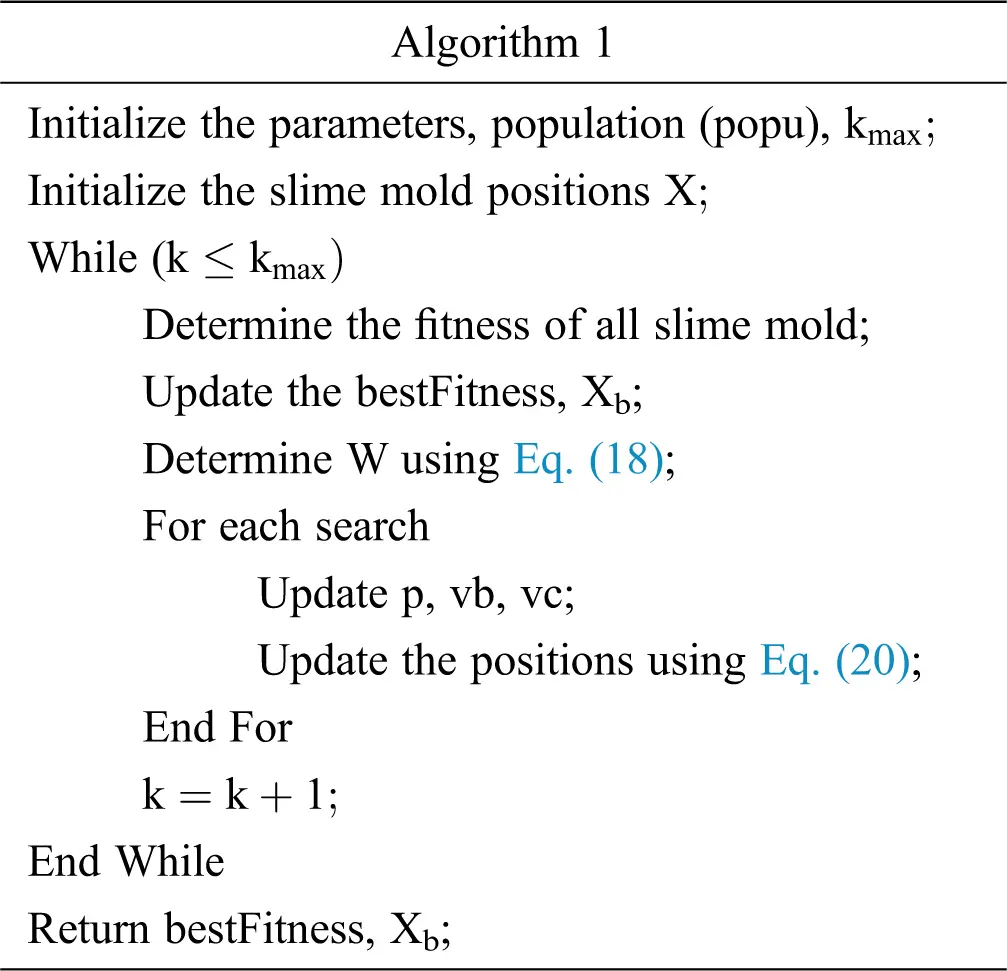Fig. 2 shows the experimental setup of the transformers in the laboratory at Taif University. The potentiometers were employed to load the transformers from 10% to 100%. The digital multimeters were used to measure currents and voltages and wattmeters for measuring electrical power.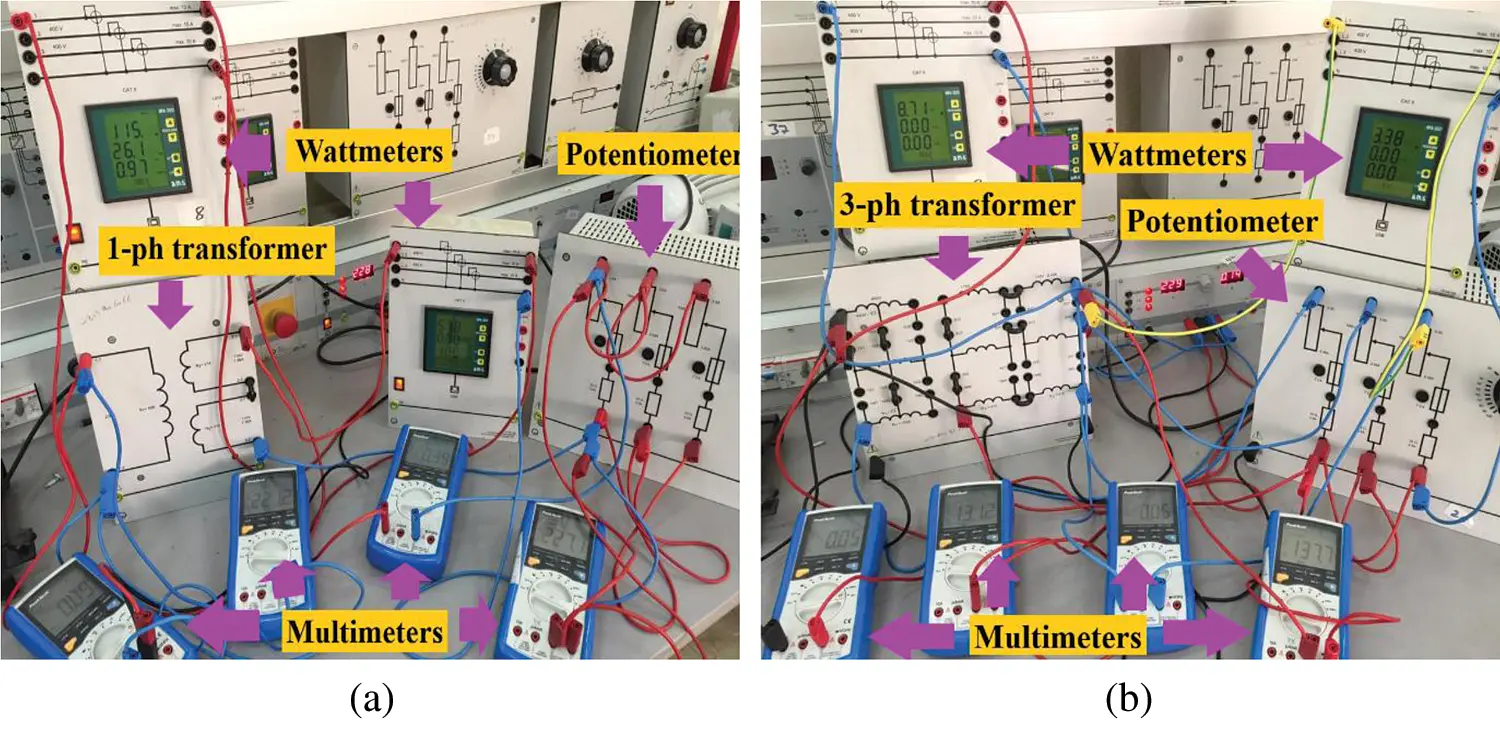Figure 2: Experimental transformer setups (a) Single-phase transformer (b) Three-phase transformer

Tabs. 2 and 3 show the transformer load test measurements. Eleven and eight measurements were obtained for the single- and three-phase transformers, respectively. The population, maximum number of iterations, and control parameters used by all the optimization algorithms (SMOA, ASO, ISA, and SFO) are listed in Tab. 4. The population and maximum number of iterations were identical for all optimizers to guarantee fairness in comparison. The control parameters of SMOA and ISA are not included because they are changed dynamically throughout the iterations. The best (fittest) values of the transformer parameters are obtained after many independent runs of SMOA, generating a minimum Fobje as these optimizers are stochastic.

Table 2: Load test of the single-phase transformer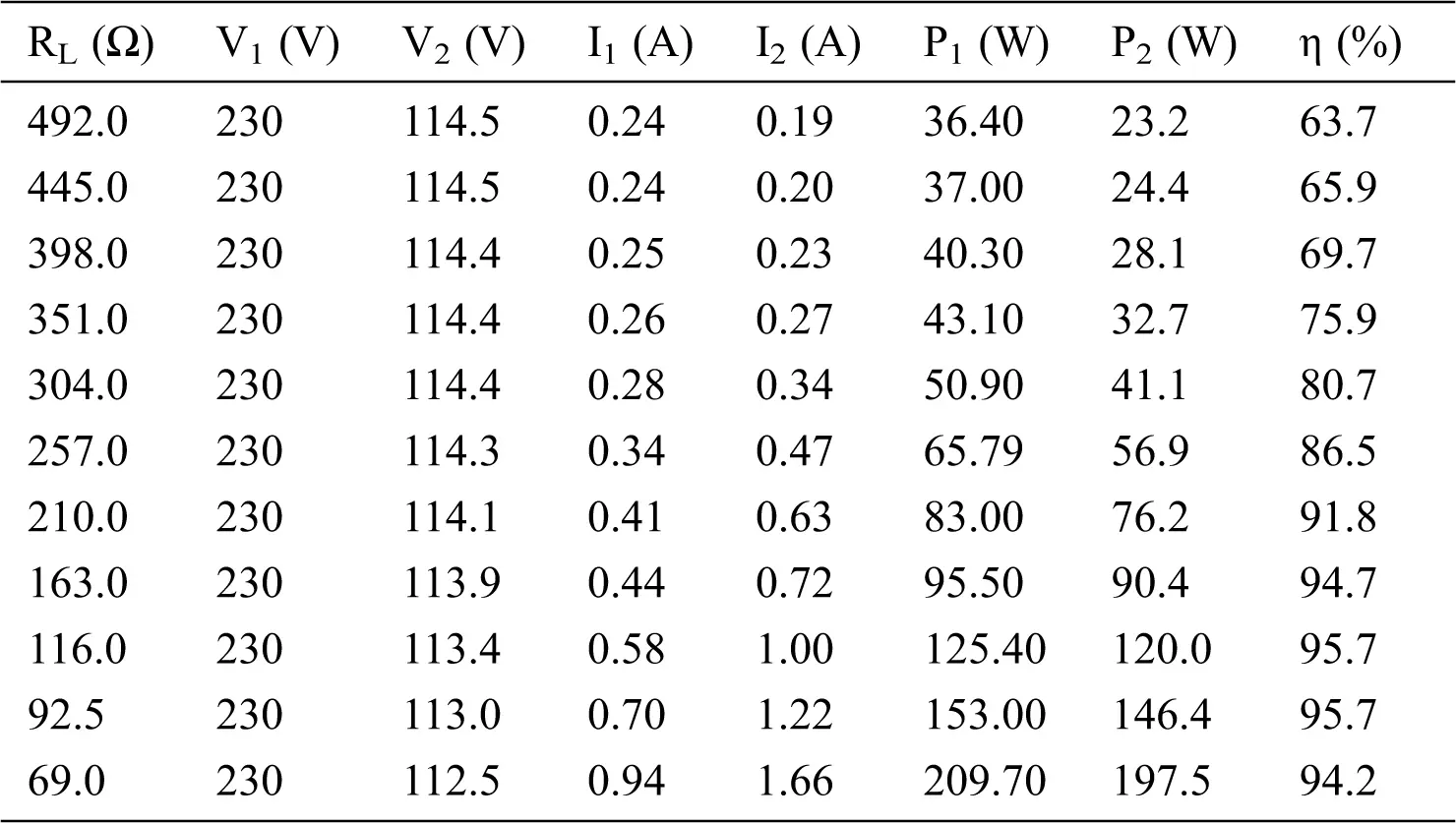Table 3: Load test of the three-phase transformer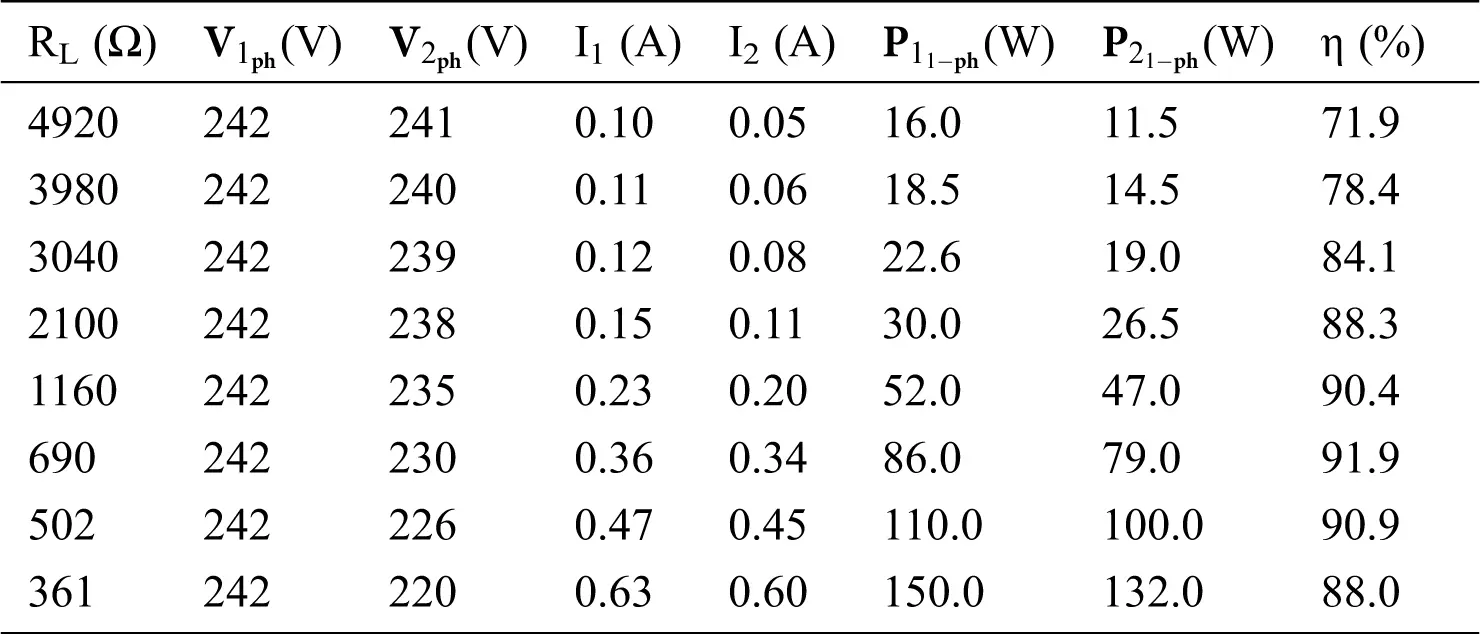Table 4: Optimizer control parameters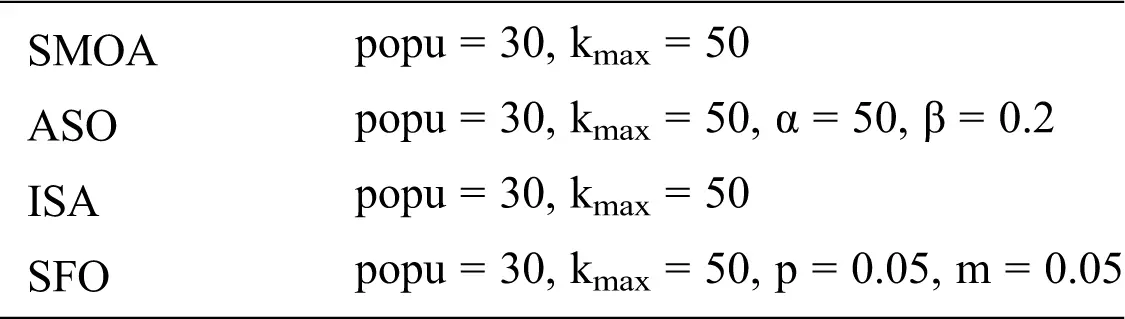After applying SMOA, the estimated transformer parameters are utilized to calculate the currents, powers, and secondary voltages via the fundamental laws of electric circuits. The smallest values of the resultant SSRE were 0.601768 and 1.16306 for the single- and three-phase transformers, respectively.

The parameters of single- and three-phase transformers are extracted using SMOA and calculated the percentage error as shown in Tabs. 5 and 6, respectively. The low error percentages demonstrate the precision of the parameters optimized via SMOA. Comparing the results from SMOA, ASO, ISA, and SFO show that the SSRE obtained using SMOA was the smallest for single- and three-phase transformers, as shown in Tabs. 7 and 8, respectively. Tab. 7 shows that the SSREs of the other methods exceed the SSRE obtained from SMOA by 0.566% for ASO, 0.006% for ISA, and 2.4085% for SFO. Tab. 8 shows the SSRE values for the other techniques were higher by 2.5536% for ASO, 0.1994% for ISA, and 5.8999% for SFO. With reference to the SSRE convergence curves in Fig. 3, SMOA had a fast and smooth convergence curve without oscillations until it obtained the optimal SSRE when compared with other techniques. Tabs. 7 and 8 show the computation time, with SMOA achieving the fastest performance for the single-phase transformer and a close second place behind ISA for the three-phase transformer. There were more measurements for the load test with the single-phase transformer, which explains the longer computation time of all the optimizers in that case.

Table 5: Optimized parameters obtained from SMOA for the single-phase transformer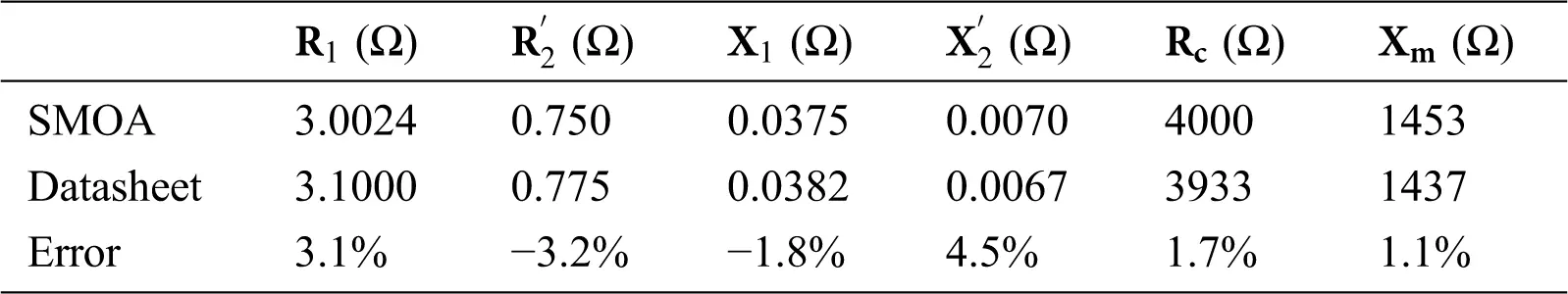Table 6: Optimized parameters obtained from SMOA for the three-phase transformer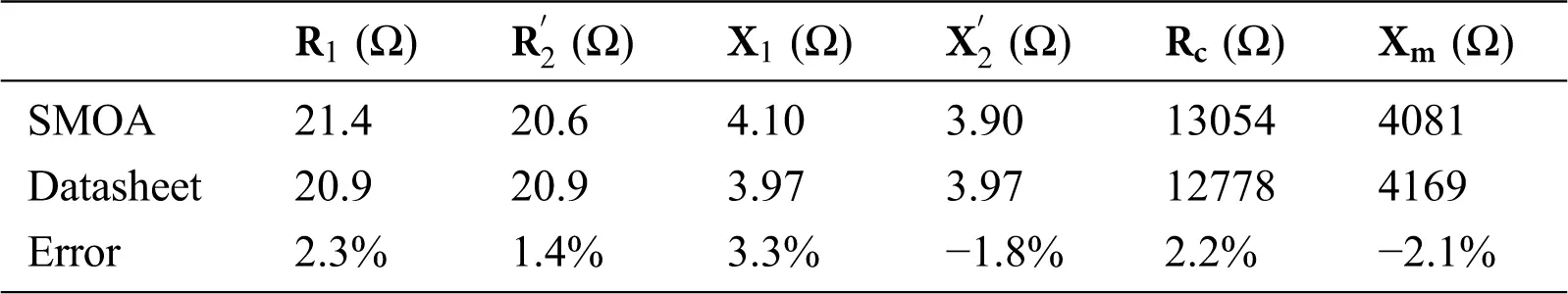Table 7: SSRE results for the single-phase transformer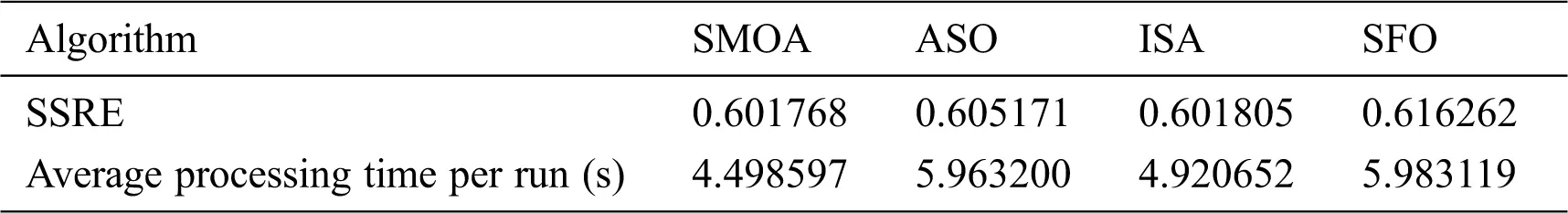Table 8: SSRE results for the three-phase transformer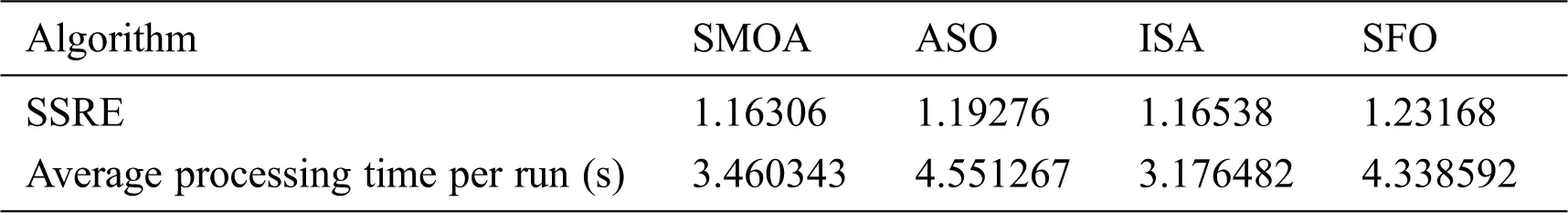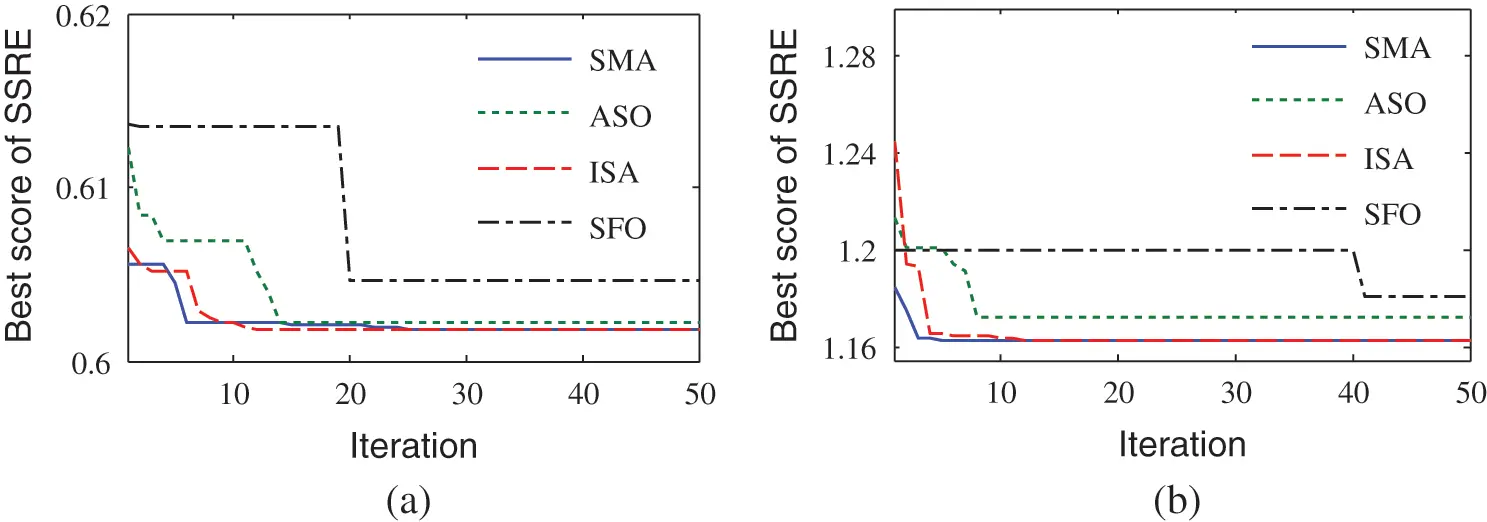Figure 3: SSRE convergence curves (a) Single-phase transformer (b) Three-phase transformer

The plots of I1-RL, I2-RL, V2-RL, P1-RL, P2-RL, and η-RL of the transformers extracted by SMOA and their measured values are displayed in Figs. 411. The closeness between the measured and calculated currents, voltages, and powers using SMOA shows the precision of our estimation method.Figure 4: Primary and secondary currents of the single-phase transformer versus load resistance (a) I1-RL plot (b) I2-RL plot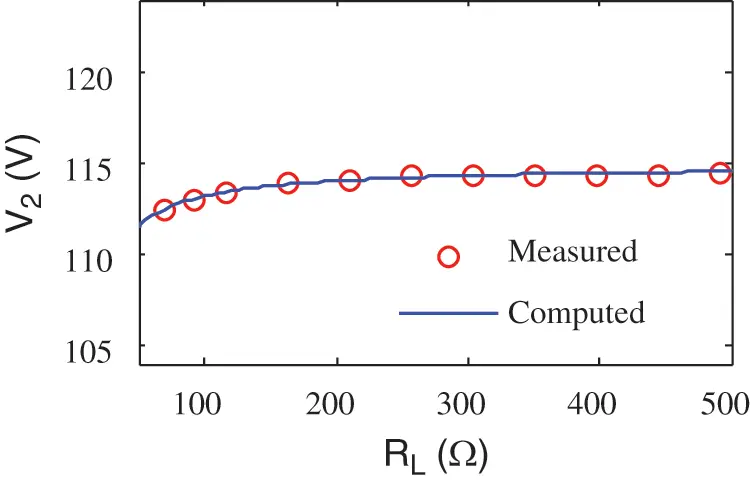Figure 5: The secondary voltage of the single-phase transformer versus load resistance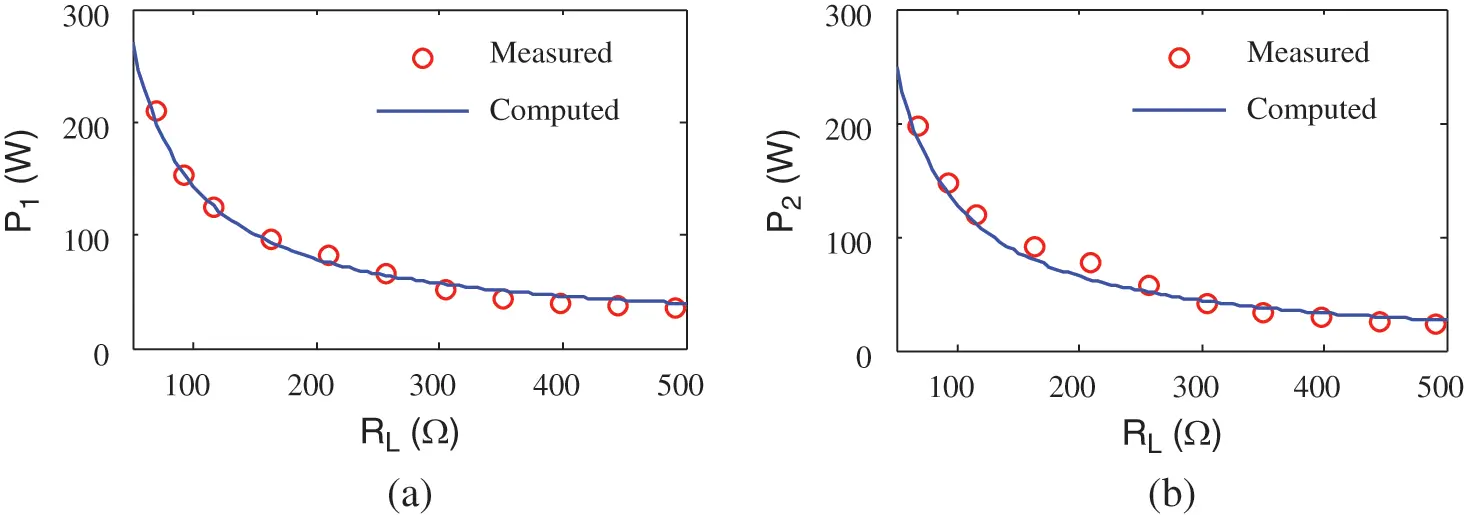Figure 6: Input and output powers of the single-phase transformer versus load resistance (a) P1-RL plot (a) P2-RL plotFigure 7: Efficiency of the three-phase transformer versus load resistance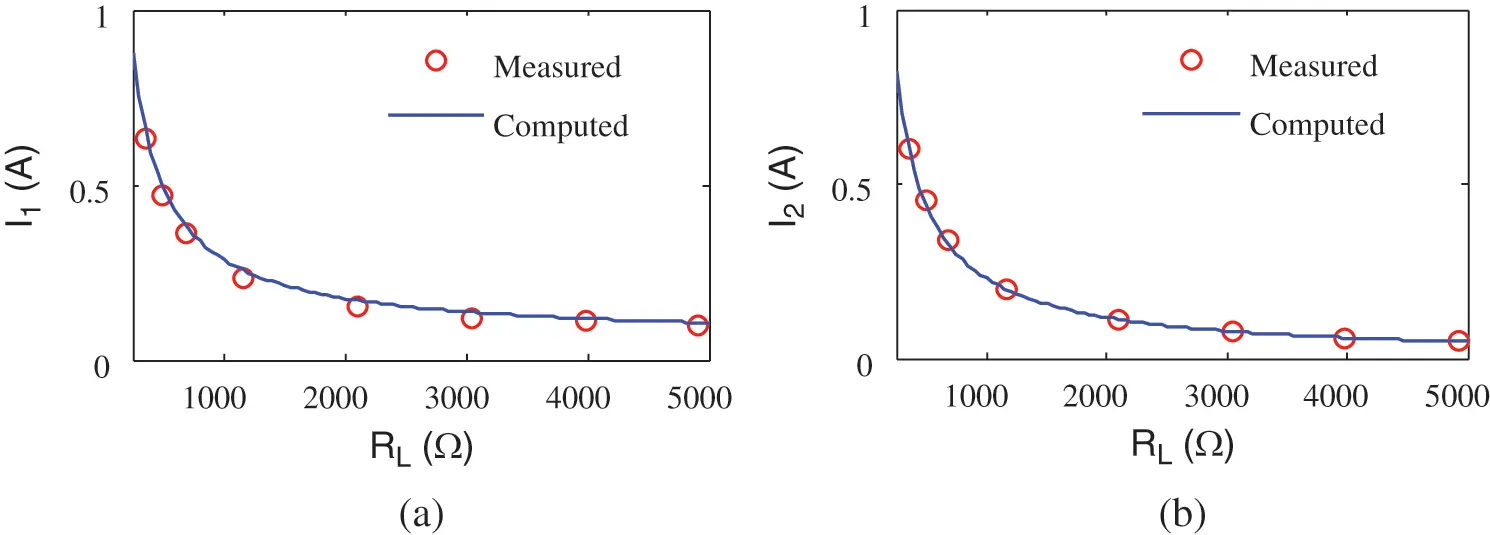Figure 8: Primary and secondary currents of the three-phase transformer versus load resistance (a) I1-RL plot (b) I2-RL plot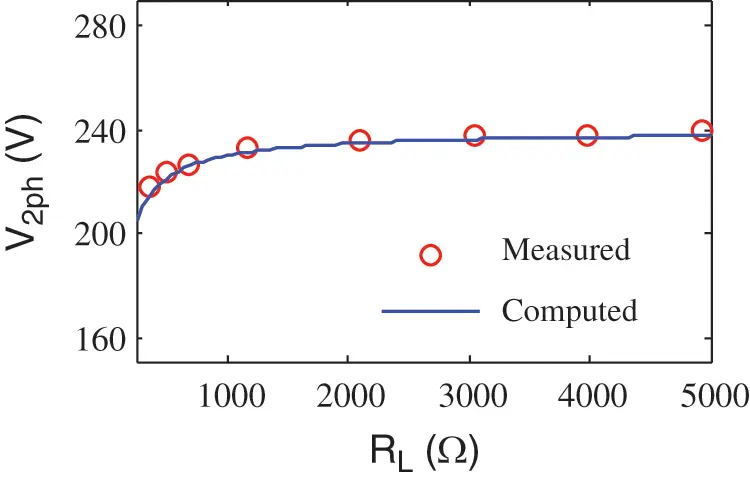Figure 9: The secondary voltage of the three-phase transformer versus load resistance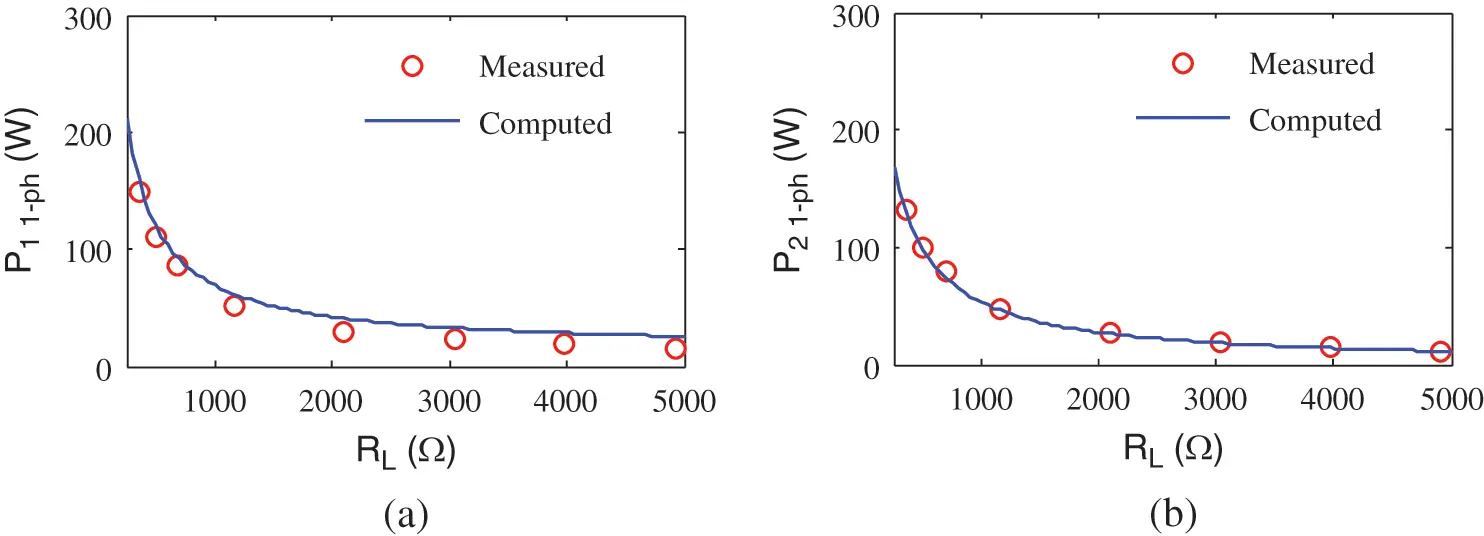Figure 10: Input and output power of the three-phase transformer versus load resistance (a) P1-RL plot (b) P2-RL plot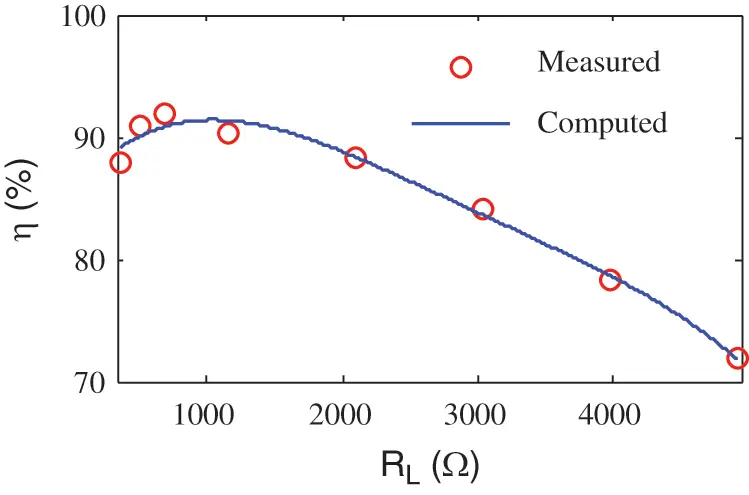Figure 11: Efficiency of the three-phase transformer versus load resistance

Tab. 9 lists the statistical results obtained when using SMOA to obtain parameters of the two transformers. The best and worst results and the standard deviation (SD) of Fobje are written. Smaller SD values emphasize the effectiveness of SMOA in identifying the unknown parameters of the two transformers.

Table 9: The statistical results of SMOA for two transformers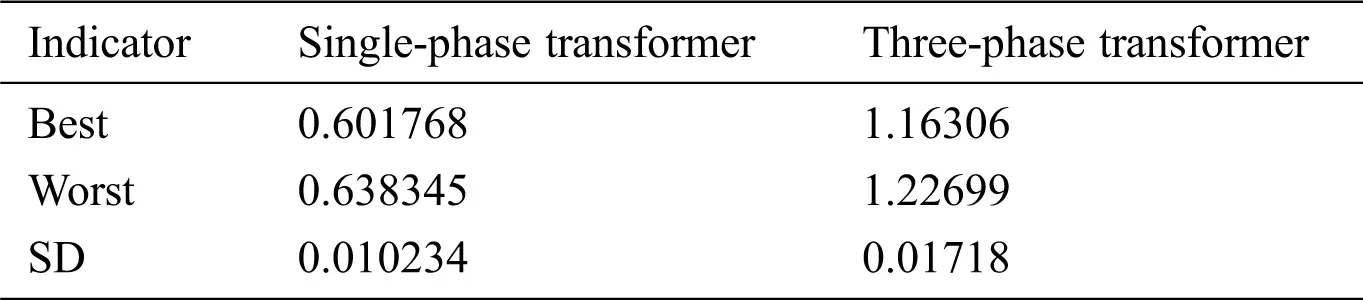5  Conclusions

Obtaining unknown parameters of the transformer equivalent circuit by load tests, is preferred because it requires less data than other methods. The use of optimization algorithms minimizes the deviations between the estimated and measured values of load test data. The authors of this paper propose using SMOA as a precise, quick, and reliable means for generating the best values of the unknown transformer parameters. Our proposed objective function seeks to minimize the sum of squared relative errors (SSREs) between the computed and measured currents, powers, and secondary voltages in a load test of the transformer. Our investigation into a test implementation of SMOA for transformer parameter estimation reveals its improved speed and accuracy compared to existing optimizers. The results show that our proposed SMOA is efficient and dependable, outperforms other approaches in terms of quicker convergence, and has superior accuracy. The authors of this paper conclude that SMOA is a precise algorithm that can be used to optimize a broad variety of parameters in the field of electrical engineering.

Acknowledgement: The authors gratefully acknowledge the approval and the support of this research study by Taif University Researchers Supporting Project number (TURSP-2020/86), Taif University, Taif, Saudi Arabia.

Funding Statement: This work was supported by Taif University Researchers Supporting Project number (TURSP-2020/86), Taif University, Taif, Saudi Arabia.

Conflicts of Interest: The authors declare that they have no conflicts of interest to report regarding the present study.

## References

1. H. D. Mehta and R. M. Patel, “A review on transformer design optimization and performance analysis using artificial intelligence techniques,” Int. Journal of Science and Research, vol. 3, no. 9, pp. 726–733, 2014.
2. A. Shintemirov, W. H. Tang and Q. H. Wu, “Transformer core parameter identification using frequency response analysis,” IEEE Trans. on Magnetics, vol. 46, no. 1, pp. 141–149, 2010.
3. E. I. Amoiralis, M. A. Tsili and A. G. Kladas, “Transformer design and optimization: A literature survey,” IEEE Trans. on Power Delivery, vol. 24, no. 4, pp. 1999–2024, 2009.
4. D. Aguglia, P. Viarouge and C. D. A. Martins, “Frequency-domain maximum-likelihood estimation of high-voltage pulse transformer model parameters,” IEEE Trans. on Industry Applications, vol. 49, no. 6, pp. 2552–2561, 2013.
5. H. Dirik, C. Gezegin and M. Özdemir, “A novel parameter identification method for single-phase transformers by using real-time data,” IEEE Trans. on Power Delivery, vol. 29, no. 3, pp. 1074–1082, 2014.
6. M. W. Q. N. Han, “Online estimation of transformer parameters based on PMU measurements,” Dianli Xitong Zidonghua/Automation of Electric Power Systems, vol. 35, no. 13, pp. 61–65, 2011.
7. S. D. Mitchell and J. S. Welsh, “Modeling power transformers to support the interpretation of frequency-response analysis,” IEEE Trans. on Power Delivery, vol. 26, no. 4, pp. 2705–2717, 2011.
8. A. Abu-Siada, M. I. Mosaad, D.Kim and M. F. El-Naggar, “Estimating power transformer high frequency model parameters using frequency response analysis,” IEEE Trans. on Power Delivery, vol. 35, no. 3, pp. 1267−1277, 2020.
9. IEEE Std, IEEE Guide for Transformer Loss Measurement. USA: IEEE, 1–49, 2010.
10. M. Ćalasan, D. Mujičić, V. Rubežić and M. Radulović, “Estimation of equivalent circuit parameters of single-phase transformer by using chaotic optimization approach,” Energies, vol. 12, no. 9, pp. 1697, 2019.
11. R. Kazemi, S. Jazebi, D. Deswal and F. De León, “Estimation of design parameters of single-phase distribution transformers from terminal measurements,” IEEE Trans. on Power Delivery, vol. 32, no. 4, pp. 2031–2039, 2017.
12. H. A. Illias, K. J. Mou and A. H. A. Bakar, “Estimation of transformer parameters from nameplate data by imperialist competitive and gravitational search algorithms,” Swarm and Evolutionary Computation, vol. 36, no. March, pp. 18–26, 2017.
13. M. I. Mossad, M. Azab and A. Abu-Siada, “Transformer parameters estimation from nameplate data using evolutionary programming techniques,” IEEE Trans. on Power Delivery, vol. 29, no. 5, pp. 2118–2123, 2014.
14. S. Subramanian and S. Padma, “Bacterial foraging algorithm based parameter estimation of three winding transformer,” Energy and Power Engineering, vol. 03, no. 02, pp. 135–143, 2011.
15. E. E. Elattar and S. K. ElSayed, “Modified JAYA algorithm for optimal power flow incorporating renewable energy sources considering the cost, emission, power loss, and voltage profile improvement,” Energy, vol. 178, no. 2, pp. 598–609, 2019.
16. N. E. Koubaa, M. Menaaa, K. Tehranib and M. Boudour, “Optimal tuning for load frequency control using ant lion algorithm in multi-area interconnected power system,” Intelligent Automation & Soft Computing, vol. 25, no. 2, pp. 279−294, 2019.
17. A. M. Agwa, “Equilibrium optimization algorithm for automatic generation control of interconnected power systems,” Przeglad Elektrotechniczny, vol. 96, no. 9, pp. 143–148, 2020.
18. Elattar E. E. and ElSayed S. K., “Probabilistic energy management with emission of renewable micro-grids including storage devices based on efficient salp swarm algorithm,” Renewable Energy, vol. 153, no. 1, pp. 23–35, 2020.
19. El-Fergany A. A., Hasanien H. M. and Agwa A. M., “Semi-empirical PEM fuel cells model using whale optimization algorithm,” Energy Conversion and Management, vol. 201, no. 31, pp. 112197, 20
20. Q. Fan, Y. Zhang and Z. Wang, “Improved teaching-learning based optimization and its application in parameter estimation of solar cell models,” Intelligent Automation & Soft Computing, vol. 26, no. 1, pp. 1−12, 20
21. A. M. Agwa, A. A. El-Fergany and H. A. Maksoud, “Electrical characterization of photovoltaic modules using farmland fertility optimizer,” Energy Conversion and Management, vol. 217, no. April, pp. 112990, 2020.
22. R. Aghmasheh, V. Rashtchi and E. Rahimpour, “Gray box modeling of power transformer windings based on design geometry and particle swarm optimization algorithm,” IEEE Trans. on Power Delivery, vol. 33, no. 5, pp. 2384–2393, 2018.
23. P. C. A. Martin, R. Vesna and D Adel, “Notes on parameter estimation for single-phase transformer,” IEEE Trans. on Power Delivery, vol. 29, no. 3, pp. 141–149, 2020.
24. Z. Yilmaz, M. Okşar and F. Başçiftçi, “Multi-objective artificial bee colony algorithm to estimate transformer equivalent circuit parameters,” Periodicals of Engineering and Natural Sciences, vol. 5, no. 3, pp. 271–277, 2017.
25. M. I. Abdelwanis, A. Abaza, R. A. El-Sehiemy, M. N. Ibrahim and H. Rezk, “Parameter estimation of electric power transformers using coyote optimization algorithm with experimental verification,” IEEE Access, vol. 8, no. March, pp. 50036–50044, 2020.
26. M. Calasan, A. Jovanovic, V. Rubezic, D. Mujicic and A. Deriszadeh, “Notes on parameter estimation for single-phase transformer,” IEEE Trans. on Industry Applications, vol. 9994, no. c, pp. 1, 2020.
27. S. Li, H. Chen, M. Wang, A. A. Heidari and S. Mirjalili, “Slime mould algorithm: A new method for stochastic optimization,” Future Generation Computer Systems, vol. 111, pp. 300–323, 2020.
28. W. Zhao, L. Wang and Z. Zhang, “A novel atom search optimization for dispersion coefficient estimation in groundwater,” Future Generation Computer Systems, vol. 91, pp. 601–610, 2019.
29. A. H. Gandomi and D. A. Roke, “Engineering optimization using interior search algorithm,” in Proc IEEE SSCI. Orlando, FL, USA, 20–26, 2014.
30. X. S. Yang, “Flower Pollination Algorithm for Global Optimization,” in Lecture Notes in Computer Science, 1st ed., vol. 7445. Heidelberg, Berlin, Germany: Springer, pp. 240–249, 2012.This work is licensed under a Creative Commons Attribution 4.0 International License, which permits unrestricted use, distribution, and reproduction in any medium, provided the original work is properly cited.